Related Articles
Python | Pandas Index.difference()
• Last Updated : 16 Dec, 2018

Python is a great language for doing data analysis, primarily because of the fantastic ecosystem of data-centric python packages. Pandas is one of those packages and makes importing and analyzing data much easier.

Pandas` Index.difference()` function return a new Index with elements from the index that are not in other.
The function automatically sorts the output if sorting is possible.

Syntax: Index.difference(other)

Parameters :
other : Index or array-like

Returns : difference : Index

Example #1: Use `Index.difference()` function to find the set difference of a given Index with an array-like object.

 `# importing pandas as pd``import` `pandas as pd`` ` `# Creating the Index``idx ``=` `pd.Index([``17``, ``69``, ``33``, ``15``, ``19``, ``74``, ``10``, ``5``])`` ` `# Print the Index``idx`

Output :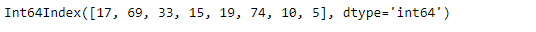Let’s find the set difference of the given Index with an array-like object

 `# find the set difference of this Index ``# with the passed array object.``idx.difference([``69``, ``33``, ``15``, ``74``, ``19``])`

Output :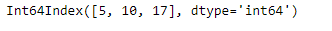As we can see in the output, the function has returned an object which contains only those values which are unique to the idx Index.

Note that the output object has its element sorted in Increasing order.

Example #2: Use `Index.difference()` function to find the set difference of two Indexes.

 `# importing pandas as pd``import` `pandas as pd`` ` `# Creating the first Index``idx1 ``=` `pd.Index([``'Jan'``, ``'Feb'``, ``'Mar'``, ``'Apr'``, ``'May'``, ``'Jun'``,``                 ``'Jul'``, ``'Aug'``, ``'Sep'``, ``'Oct'``, ``'Nov'``, ``'Dec'``])`` ` `# Creating the second Index``idx2 ``=` `pd.Index([``'May'``, ``'Jun'``, ``'Jul'``, ``'Aug'``])`` ` `# Print the first and second Index``print``(idx1, ``"\n"``, idx2)`

Output :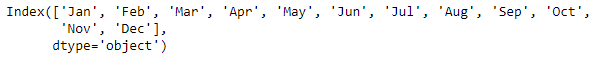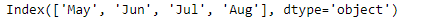Now, let’s find the set difference between two Indexes.

 `# to find the set differnece``idx1.difference(idx2)`

Output :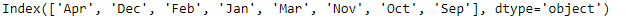The function has returned the set difference of idx1 and idx2. It contains only those values which are unique to idx1 Index. Note that the output is not sorted.

Attention geek! Strengthen your foundations with the Python Programming Foundation Course and learn the basics.

To begin with, your interview preparations Enhance your Data Structures concepts with the Python DS Course.

My Personal Notes arrow_drop_up